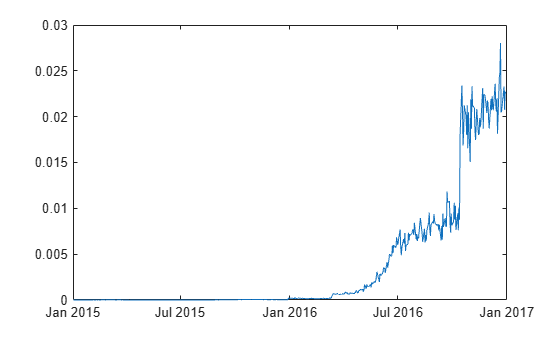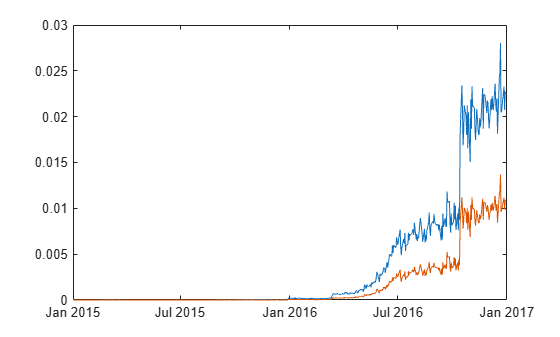mertonByTimeSeries

Estimate default probability using time-series version of Merton model

Description

example

[PD,DD,A,Sa] = mertonByTimeSeries(Equity,Liability,Rate) estimates the default probability of a firm by using the Merton model.

example

[PD,DD,A,Sa] = mertonByTimeSeries(___,Name,Value) adds optional name-value pair arguments.

Examples

collapse all

Dates     = MertonDataTS.Dates;
Equity    = MertonDataTS.Equity;
Liability = MertonDataTS.Liability;
Rate      = MertonDataTS.Rate;

Compute the default probability by using the time-series approach of Merton's model.

[PD,DD,A,Sa] = mertonByTimeSeries(Equity,Liability,Rate);
plot(Dates,PD)Dates     = MertonDataTS.Dates;
Equity    = MertonDataTS.Equity;
Liability = MertonDataTS.Liability;
Rate      = MertonDataTS.Rate;

Compute the plot for the default probability values by using the time-series approach of Merton's model. You compute the PD0 (blue line) by using the default values. You compute the PD1 (red line) by specifying an optional Drift value.

PD0 = mertonByTimeSeries(Equity,Liability,Rate);
PD1 = mertonByTimeSeries(Equity,Liability,Rate,'Drift',0.10);
plot(Dates, PD0, Dates, PD1)Input Arguments

collapse all

Market value of the firm’s equity, specified as a positive value.

Data Types: double

Liability threshold of the firm, specified as a positive value. The liability threshold is often referred to as the default point.

Data Types: double

Annualized risk-free interest rate, specified as a numeric value.

Data Types: double

Name-Value Arguments

Specify optional comma-separated pairs of Name,Value arguments. Name is the argument name and Value is the corresponding value. Name must appear inside quotes. You can specify several name and value pair arguments in any order as Name1,Value1,...,NameN,ValueN.

Example: [PD,DD,A,Sa] = mertonByTimeSeries(Equity,Liability,Rate,'Maturity',4,'Drift',0.22,'Tolerance',1e-5,'NumPeriods',12)

Time to maturity corresponding to the liability threshold, specified as the comma-separated pair consisting of 'Maturity' and a positive value.

Data Types: double

Annualized drift rate, expected rate of return of the firm's assets, specified as the comma-separated pair consisting of 'Drift' and a numeric value.

Data Types: double

Number of periods per year, specified as the comma-separated pair consisting of 'NumPeriods' and a positive integer. Typical values are 250 (yearly), 12 (monthly), or 4 (quarterly).

Data Types: double

Tolerance for convergence of the solver, specified as the comma-separated pair consisting of 'Tolerance' and a positive scalar value.

Data Types: double

Maximum number of iterations allowed, specified as the comma-separated pair consisting of 'MaxIterations' and a positive integer.

Data Types: double

Output Arguments

collapse all

Probability of default of the firm at maturity, returned as a numeric.

Distance-to-default, defined as the number of standard deviations between the mean of the asset distribution at maturity and the liability threshold (default point), returned as a numeric.

Value of firm's assets, returned as a numeric value.

Annualized firm's asset volatility, returned as a numeric value.

collapse all

Merton Model for Time Series

In the Merton model, the value of a company's equity is treated as a call option on its assets, and the liability is taken as a strike price.

Given a time series of observed equity values and liability thresholds for a company, mertonByTimeSeries calibrates corresponding asset values, the volatility of the assets in the sample's time span, and computes the probability of default for each observation. Unlike mertonmodel, no equity volatility input is required for the time-series version of the Merton model. You compute the probability of default and distance-to-default by using the formulae in Algorithms.

Algorithms

Given the time series for equity (E), liability (L), risk-free interest rate (r), asset drift (μA), and maturity (T), mertonByTimeSeries sets up the following system of nonlinear equations and solves for a time series asset values (A), and a single asset volatility (σA). At each time period t, where t = 1...n:

$\begin{array}{l}{A}_{1}=\left(\frac{{E}_{1}+{L}_{1}{e}^{-{r}_{1}{T}_{1}}N\left({d}_{2}\right)}{N\left({d}_{1}\right)}\right)\\ {A}_{t}=\left(\frac{{E}_{t}+{L}_{t}{e}^{-{r}_{t}{T}_{t}}N\left({d}_{2}\right)}{N\left({d}_{1}\right)}\right)\\ ...\\ {A}_{n}=\left(\frac{{E}_{n}+{L}_{n}{e}^{-{r}_{n}{T}_{n}}N\left({d}_{2}\right)}{N\left({d}_{1}\right)}\right)\end{array}$

where N is the cumulative normal distribution. To simplify the notation, the time subscript is omitted for d1 and d2. At each time period, d1, and d2 are defined as:

${d}_{1}=\frac{\mathrm{ln}\left(\frac{A}{L}\right)+\left(r+0.5{\sigma }_{A}^{2}\right)T}{{\sigma }_{A}\sqrt{T}}$

${d}_{2}={d}_{1}-{\sigma }_{A}\sqrt{T}$

The formulae for the distance-to-default (DD) and default probability (PD) at each time period are:

$DD=\frac{\mathrm{ln}\left(\frac{A}{L}\right)+\left({\mu }_{A}-0.5{\sigma }_{A}^{2}\right)T}{{\sigma }_{A}\sqrt{T}}$

$PD=1-N\left(DD\right)$

 Zielinski, T. Merton's and KMV Models In Credit Risk Management.

 Loeffler, G. and Posch, P.N. Credit Risk Modeling Using Excel and VBA. Wiley Finance, 2011.

 Kim, I.J., Byun, S.J, Hwang, S.Y. An Iterative Method for Implementing Merton.

 Merton, R. C. “On the Pricing of Corporate Debt: The Risk Structure of Interest Rates.” Journal of Finance. Vol. 29. pp. 449–470.